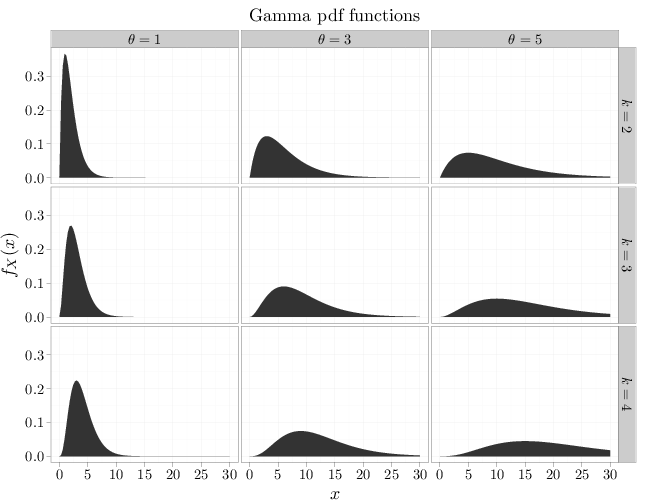## Probability

### The Analysis of Data, volume 1

Important Random Variables: The Gamma and Chi-Squared Distributions

## 3.10. The Gamma and $\chi^2$ Distributions

Definition 3.10.1. The gamma function is $\Gamma(x) =\int_0^{\infty} t^{x-1}\exp(-t)\, dt, \qquad x>0.$

Example F.2.5 shows, using integration by parts, that $\Gamma(n)=(n-1)!$ for positive integers $n$. The gamma function, however, is also defined for real numbers that are not integers.

Definition 3.10.2. The gamma RV, $X\sim \text{Gam}(k,\theta)$, where $k,\theta\geq 0$, has the following pdf: \begin{align} f_X(x) = \begin{cases} x^{k-1} \theta^{-k} \exp(-x/\theta)/\Gamma(k) & x,k,\theta\geq 0\\ 0 &\text{otherwise}\end{cases}. \end{align}

The gamma function in the denominator of the pdf ensures that the pdf integrates to 1: \begin{align} \int_0^{\infty} x^{k-1} \exp(-x/\theta)\,dx= \theta\theta^{k-1} \int_0^{\infty} z^{k-1} \exp(-z)\,dz = \theta^k\Gamma(k). \end{align}

Proposition 3.10.1. The mgf of the gamma distribution is $m(t) = (1-\theta t)^{-k}$, for $t <1/\theta$.
Proof. Defining $y=(1/\theta-t)x$, \begin{align*} m(t) &= \E(\exp(tX)) = \int_0^{\infty} x^{k-1} \frac{\exp(-x/\theta)}{\Gamma(k)\theta^k}\exp(tx) \, dx \\&=\frac{1}{\Gamma(k)\theta^k} \int_0^{\infty} \left(\frac{y}{1/\theta-t} \right)^{k-1} \exp(-y) \, dy \frac{1}{1/\theta-t}\\ &=\frac{1}{\Gamma(k) (\theta(1/\theta-t)^{k}} \int_0^{\infty} y^{k-1}\exp(-y) \, dy = \left(1-\theta t\right)^{-k}. \end{align*}
Proposition 3.10.2. If $X_i\sim \text{Gam}(k_i,\theta)$, $i=1m\ldots,n$ are independent RVs, then $\sum_{i=1}^n X_i \,\sim\, \text{Gam}\left(\sum_{i=1}^n k_i,\theta\right).$
Proof. This follows from the fact that the mgf of a sum of RVs is the product of their mgfs: $\prod (1-\theta t)^{-k_i}=(1-\theta t)^{\sum k_i}$.

Using the mgf, we can compute the expectation and variance of $X\sim \text{Gam}(k,\theta)$: \begin{align*} \E(X) &=m'(0) = k\theta (1-\theta t)^{-k-1}\Big |_{t=0} =k\theta\\ \E(X^2) &= m''(0)= k\theta (-k-1)(1-\theta t)^{-k-2}\Big |_{t=0}(-\theta)=k\theta^2(k+1) \\ \Var(X) &= k\theta^2(k+1) = k^2\theta^2= k\theta^2. \end{align*}

The non-constant parts of the pdf above (the parts that depend on $x$) include two multiplied terms: a decaying exponential $\exp(-x/\theta)$ and a polynomial $x^{k-1}$. As $x$ increases, the polynomial term is monotonic increasing and the exponential term is monotonic decreasing. For large $x$ the exponential decay is stronger than the polynomial growth (see Section B.5), implying that the pdf may be monotonic increasing for a while, but eventually decays to zero as $x\to\infty$. The shape of the pdf is generally unimodal, as in the case of the Gaussian distribution. Since the support is $[0,\infty)$, the pdf is necessarily asymmetric.

The R code below graphs the pdf of multiple gamma RVs with different parameter values.

x = seq(0, 30, length = 100)
y1 = dgamma(x, 2, scale = 1)
y2 = dgamma(x, 2, scale = 3)
y3 = dgamma(x, 2, scale = 5)
y4 = dgamma(x, 3, scale = 1)
y5 = dgamma(x, 3, scale = 3)
y6 = dgamma(x, 3, scale = 5)
y7 = dgamma(x, 4, scale = 1)
y8 = dgamma(x, 4, scale = 3)
y9 = dgamma(x, 4, scale = 5)
D = data.frame(probability = c(y1, y2, y3, y4, y5,
y6, y7, y8, y9))
D$x = x D$k[1:300] = "$k=2$"
D$k[301:600] = "$k=3$" D$k[601:900] = "$k=4$"
D$theta[1:100] = "$\\theta=1$" D$theta[101:200] = "$\\theta=3$"
D$theta[201:300] = "$\\theta=5$" D$theta[301:400] = "$\\theta=1$"
D$theta[401:500] = "$\\theta=3$" D$theta[501:600] = "$\\theta=5$"
D$theta[601:700] = "$\\theta=1$" D$theta[701:800] = "$\\theta=3$"
D$theta[801:900] = "$\\theta=5$" qplot(x, probability, data = D, geom = "area", facets = k ~ theta, xlab = "$x$", ylab = "$f_X(x)$", main = "Gamma pdf functions")Definition 3.10.3. The chi-squared RV,$X\sim\chi^2_r$, where$r\in\mathbb{N}$, is a gamma RV with parameters$k=r/2,\theta=2$. The parameter$r$is called the degrees of freedom. Corollary 3.10.1. The mgf of the chi-squared distribution$\chi^2_r$is$m(t)=(1-2t)^{-r/2}$. The primary motivation of the chi-squared distribution is the following observation. Proposition 3.10.2. If$Z^{(1)},\ldots,Z^{(n)}$are independent$N(0,1)$RVs then $\sum_{i=1}^n (Z^{(i)})^2\,\,\sim\,\,\chi^2_n.$ Proof. Consider first the case$n=1$. The mgf of$(Z^{(1)})^2is \begin{align*} \E\left(e^{tZ^2}\right) &= \int_{-\infty}^{+\infty} e^{tz^2} (2\pi)^{-1/2} e^{-z^2/2} dz = \int_{-\infty}^{+\infty} (2\pi)^{-1/2} e^{-(1-2t)z^2/2} dz \\&= \frac{1}{(1-2t)^{1/2}} \int_{-\infty}^{+\infty} \frac{e^{-z^2/(2(1-2t)^{-1})}}{\sqrt{2\pi} (1-2t)^{-1/2}} dz = \frac{1}{(1-2t)^{1/2}} \cdot 1 \end{align*} which is the mgf of\chi^2_1$. In the case$n>1$, the mgf of the sum is the product of$n$mgfs of chi-squared RVs with one degree of freedom (see Proposition 4.8.1). This product is$\prod_{i=1}^n(1-2t)^{-1/2}=(1-2t)^{-n/2}$, which is the mgf of a$\chi^2_r\$ RV.